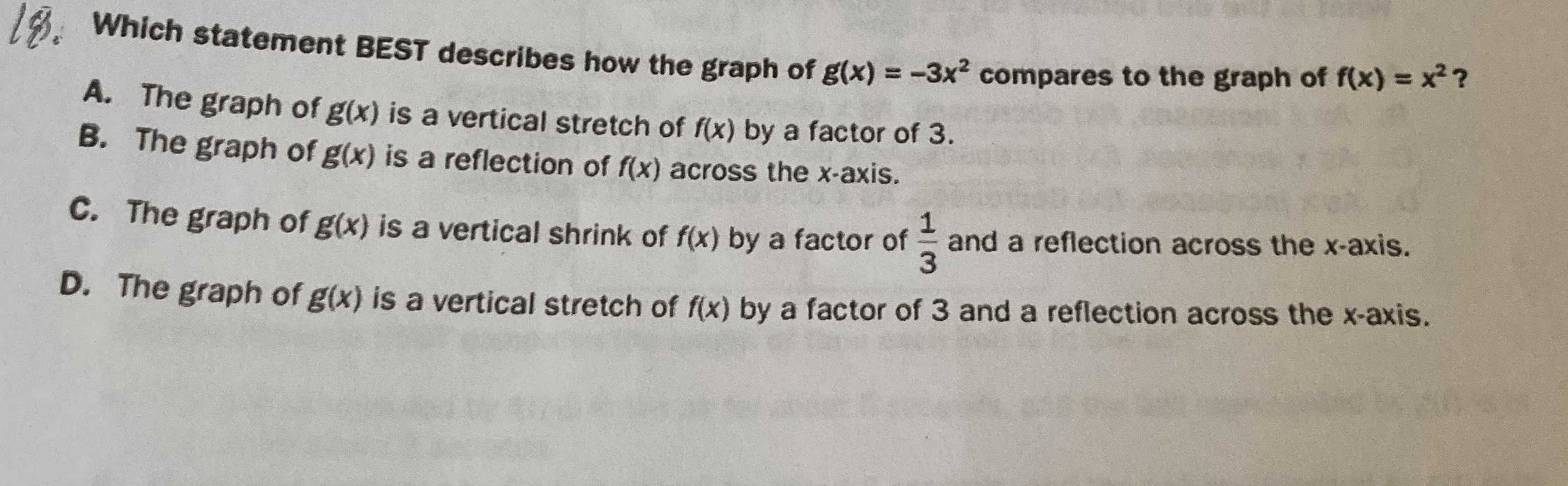### ¿Todavía tienes preguntas de matemáticas?

Pregunte a nuestros tutores expertos
Algebra
Pregunta19. Which statement BEST describes how the graph of $$g ( x ) = - 3 x ^ { 2 }$$ compares to the graph of $$f ( x ) = x ^ { 2 }$$ ?

A. The graph of $$g ( x )$$ is a vertical stretch of $$f ( x )$$ by a factor of $$3$$ .

B. The graph of $$g ( x )$$ is a reflection of $$f ( x )$$ across the $$x$$ -axis.

C. The graph of $$g ( x )$$ is a vertical shrink of $$f ( x )$$ by a factor of $$\frac { 1 } { 3 }$$ and a reflection across the $$x$$ -axis.

D. The graph of $$g ( x )$$ is a vertical stretch of $$f ( x )$$ by a factor of $$3$$ and a reflection across the $$x$$ -axis.